# Similar experiments were performed by a number of researchers in our time . The first reasonable explanation of the results was apparently suggested by Oliver Lodge: this invisible part of the world operates at higher frequencies than the ones in the world we are able to sense. Many investigators support this idea. However, the theoretical basis regarding such a world is absent. Most authors think of this world as a "spirit" world.

A substantial step in understanding the universe was taken by Ronald Pearson . Pearson's theory is based on the idea that when two kinds of primary particles are present, collisions and the exchange of energy between them cause the creation of a neuron-like structure. This theory has opened a new field for modeling and explaining so-called "paranormal" phenomena. The wave nature of our world, which is closely linked to Pearson's theory, can be used to explain the existence of parallel worlds. Such parallel worlds are material to the same degree as our sensed world is. This means that they have the same wave nature with the same (or similar) physical laws.

With this idea as a starting point, one can develop a physical, quantum mechanics model of the higher frequency, parallel (ethereal) world that is usually invisible for us.

## The Model

Based on experimental evidence, the following main principles were used in the developing the physical model:

1. The ethereal world is real and independent of our minds;

2. It exists in our "SPACE" and in our "TIME";

3. The ethereal world has the wave (field) nature;

4. Ethereal bodies have a complex internal structure and consist of small elementary wave-particles;

5. The geometrical sizes and energy values of etheric bodies are of the same order as ones in our part of the universe;

6. The ethereal world is usually invisible for us and for our devices.

According to Pearson's theory, all energy in the universe is kinetic, and particles which have a non-zero rest mass consist of photon-like (or neutrino-like) particles with zero rest mass moving at the speed of light. The same conclusion was made by G. Shipov who developed the theory of the physical vacuum .

Let us consider the structure of a photon. A photon is a moving wave package consisting of N harmonic waves with close wavelengths, in a narrow interval of λ1 to λN, (or, equivalently, with close wave numbers k=2π/λ, from k1 to kN), with amplitudes A1, with total energy ε. Photons move at a constant velocity named the speed of light, c. This speed depends upon the properties of the medium in which the photon moves. For a given speed, c, the corresponding frequencies of wave harmonics can be found:

νi=ci=c×ki/2π  and  ωi=c×ki , i=1,...N (1)

The energy of the wave package can be found as follows: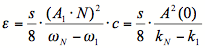(2)

where s is the cross-section area of the wave package, A(0)=A1N is the amplitude of the package. As it can be seen from eq.(2), the energy of the wave package depends on geometrical parameters (ki and A) and does not depend on the speed, c, and frequencies, ωi. In the world with a certain speed of light, c, the energy of the package can be rewritten in the form:(3)

where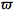is the average, carrying frequency,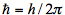, where h is Planck's constant,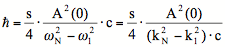(4)

Sinceis constant for all average frequencies of photons (as was found in experiments), it should be concluded that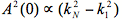for all photons, of any kind. Another consequence of eq.(4) is that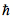is inversely proportional to the speed of light, c, and that their product is constant:(5)

It should be noted that this product is very important because it determines the elementary electrical charge, e:(6)

Let us now assume that the speed of light depends on structure and properties of the medium (ether). According to Pearson's theory, the ether (or i-ther) is an inhomogeneous medium consisting of two kinds of particles moving with different velocities.

In this case the wave package can be constructed from harmonic waves spreading in a sub-space of a certain type of particle. Spreading speeds of different wave packages can be different (in the same manner as speeds of sound waves in a solid consisting of several types of atoms). So we may assume that at least two kinds of photons exist, and the corresponding speeds of light, c1 and c2, may differ substantially: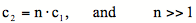(7)

The geometry of the wave packages (an amplitude A(0) and bordering wave lengths, λ1 and λN) are determined by the geometry of  the ether network and can be assumed to be similar. In this case the energy and the size of packages (average wavelengths) are the same, but the Planck's constants are different:(8)

So, Planck's constant decreases with an increase in the speed of light. The carrying frequency of the wave package increases accordingly: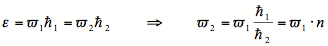(9)

According to the famous equation: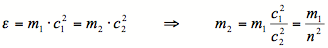(10)

photon mass is in inverse proportion to the square of the speed of light. Since the momentum of the photon is p=ε/c, increasing the speed of light leads to a decrease in the momentum: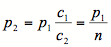(11)

Thus, we have two kinds of photons, with the same range of energies and sizes, but with substantially different frequencies, momentums and masses, and moving with different speeds.

What now can be said about a particle consisting of photons or photon-like particles? Certainly, for such particles, previous statements about decreasing masses and increasing frequencies remain true. Take, for example, an electron with a non-zero resting mass. According to Pearson's theory, this mass is a sum of kinetic masses of photon-like particles forming this electron. Therefore its resting mass will obey the law of eq.(10). The resting energy of this electron, ε0e, is the same in both worlds as well as its Compton's radius:(12)

According to quantum mechanics, every moving particle has wave properties. The corresponding wavelength is determined by the de Broglie relation λ=h/p=h/mv, where m is the mass of the particle and v is its velocity. The frequency of the particle, f, is given by the relation f=Wkin/h, where Wkin is the kinetic energy (usual meaning) of the particle.

Wave behavior of electrons manifests itself, for example, in such phenomena as diffraction of electrons passing through metal film. However, these are probability waves, and diffraction patterns are the result of statistics. Statistical behavior of particles (described by quantum mechanics) is connected with their space extent: a particle can be considered as a wave package of harmonic waves. This package can be described as a quantum liquid drop with infinite degrees of freedom [6,7]. By the way, all particles have a field, wave nature, but real lengths of harmonic waves forming the particle are determined by relations similar to eq.(12) and not by the de Broglie relation.

Assuming that typical energies of free elementary particles in both cases have the same order we may conclude that typical frequencies of particles in the second world (we'll name this the ethereal world) are much higher than ones in our first, "physical" world: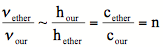(13)

This is true for all elementary particles (electrons, protons, neutrino, etc.): typical frequencies of the moving elementary particles increase proportionally to the speed of light and their masses decrease proportionally to the square of the light speed.

New electrons, protons and neutrons may form new atoms in the second world. Since the elementary charge, e, does not change (see eq.(6)), the geometrical structure of atoms is conserved. For example, Bohr radius, which determines the hydrogen atom size, does not change: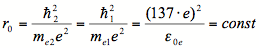(14)

This is true also for the Bohr magneton, which determines the magnetic properties of elementary particles and atoms: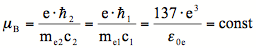(15)

Energy levels and the radii of the circular orbits of the electron in the hydrogen atom are computed by the formulas: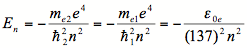(16)(17)

As can be seen, they are the same in both worlds.

An atom can absorb a discrete portion (quantum) of electromagnetic energy (photon) equal to the difference between the levels of energy in spectrum (16) if an electron "jumps" from a closer orbit to a farther one. An excited atom radiates a photon when an electron returns to a closer orbit from a farther one. The magnitude of the emitted or absorbed quantum of energy,ωk, is given by the condition: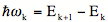(18)

The same conditions hold valid for many-electron atoms.

So, the energy spectrum of electromagnetic waves - photons - emitted and absorbed by atoms in our world and in the supposed ethereal one is the same. But the frequency spectrums are different: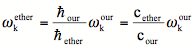(19)

This is most likely the main reason for the fact that we cannot sense or record the electromagnetic waves from the ethereal world with our devices: photons emitted by ethereal atoms have appropriate energies, but much higher frequencies. An atom as an oscillatory system can absorb energy of the waves with resonance frequencies only.

This means that our atoms cannot absorb photons emitted by analogous ethereal atoms. On the other hand, ethereal photons with appropriate (low) frequencies (created, for example, during braking of ethereal electrons) may be absorbed by our atoms, but they have too little energy (because of small Planck's constant) in comparison with "our" photons, and transfer less momentum than "our" photons by a factor of n2.  This makes it difficult to recognize them in the total signal.

Thus, the parallel, ethereal world has the following features:

1. The speed of light is much higher, than in our world, eq.(7);

2. Typical frequencies of elementary particles (photons, electrons, protons, neutrino, etc.,) are much higher than the corresponding ones in our world, eqs.(9), (13), (19);

3. Planck's constant is much smaller, eq.(8);

4. Masses of elementary particles as well as atoms are much smaller, eq.(10);

5. Elementary charge is the same, eq.(6);

6. The structure of atoms: size, geometry and energy spectrum of atoms are the same as in our world;

7. Ethereal solid, liquid and gaseous matter can be formed from ethereal atoms in the same manner as in our world;

8. Ethereal photons as well as the other ethereal elementary particles are invisible to us because of their high frequencies; this is true also for ethereal atoms and for solid matter built from these atoms.

The last statement should be considered the most carefully because of its great importance. It is based on the assumption that the world has a fully wave nature, and all elementary particles are constructed from photon-like or neutrino-like particles with zero rest mass. In this case, two different wave objects may occupy the same place.

They influence each other, leading to an interference picture, only in the case where their frequencies are similar. In the quantum mechanics language the condition for mutual influence of atoms is the appropriate frequencies of the electromagnetic fields carried by the photons which are absorbed and emitted by those atoms.

In ordinary life we feel surrounding things due to electromagnetic interaction between our own atoms (in our eyes, skin, and neurons, for example) and photons emitted by atoms of surrounding bodies. Since our atoms cannot absorb photons emitted by ethereal atoms, the ethereal world is invisible to us.

The second type of interaction we have with surrounding bodies is gravitational. We feel the attraction of the Earth, and most likely the main reason for our need of energy is the necessity to overcome the force of gravitation.

It is interesting to note that the evolution of life on Earth can be considered as a history of struggle for food that, in turn, is required for the energy needed to overcome gravitation! All our digestive, blood and respiratory systems are needed to provide energy for muscle systems - again - to overcome gravitation!

In the supposed ethereal world, the gravitational forces are very weak. Actually, the gravitational force between two bodies,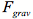, is proportional to the product of their masses, m1m2, and in the ethereal world it will be n4 times less than the force between analogous bodies in the our world. For example, if n=100,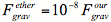.  It means that living etherians, if they exist, don't need energy to overcome gravitational attraction.

What would life be like in the ethereal world? We may suppose that etherians have no problems with food because they don't need nearly as much energy as Earth people. They may possibly receive appropriate chemical energy (mainly for brain and nervous activity) immediately from electromagnetic fields (fluxes of ethereal photons) and neutrino flows.

If so, they don't need digestive and blood systems, nor a respiratory system. They can move very quickly because of the absence of gravitational forces and atmosphere resistance, small mass and high light velocity. So, they have no problems with territory and do not struggle for their existence. At least, not in the same way as in our world.

Can we interact with this world? Might there exist a number of parallel worlds with different constants c and? These are interesting questions...

## Parallel Ethereal World and the Principle of Relativity

According to the Special Theory of Relativity, we live in a 4-dimensional world in which three coordinates are spatial, and the forth one is connected with time, t, and should be taken in the form (ict), where c is the light velocity and i is the imaginary unit. In this space every material body or system has a 4-dimensional energy-momentum vector, (cpx, cpy, cpz, imc2). The square of this vector is invariant for all inertial (that is, non-accelerated) frames of reference:(20)

If, for example, a frame of reference is connected with the particle moving along axis X with the velocity v, coordinates and momentums in this new reference are connected with ones in the initial frame of reference by the Lorentz relations: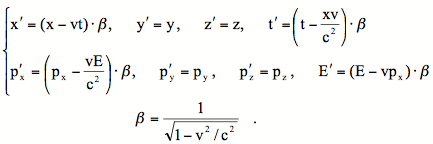(21)

The speed of light, c, is the same for all such frames of reference, independent of the motion of the observer, and it is the maximum possible velocity for all material bodies in our world.

Special theory of relativity does not consider, however, the possibility of relative motion along the forth, time-axis. Our world can be considered as moving along this axis with the speed of light.

Let us introduce a new frame of reference which moves along the time-axis with the speed c1. Coordinate transformation which conserves a square of energy-momentum vector is given by:(22)

The last equation requires also the change of masses: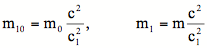(23)

that exactly coincides with eqs.(10). In this case the square of energy-momentum vector is invariant of the transformation (22):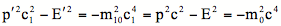(24)

Velocities of a body in the new and the old frames of reference are connected by the relation: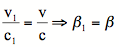(25)

So, we obtained the same results as previously, eqs.(10), (11). The new result is the change in the rate of time: with increasing the light velocity the rate of time decreases. But what is the physical meaning of time?

We use time to characterize: a) space transference of material objects; b) the rate of processes such as transformations of elementary particles, nuclear and chemical reactions. Transference in space is characterized by the product (v×t). According to eqs. (22) and (25), this product does not change: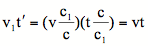(26)

As about nuclear and chemical reactions as well as transformations of elementary particles, their rates, apparently, are also connected with the light velocity in the given frame of reference, namely, they are proportional to it. If K is the rate of a certain reaction in the system with the light velocity c, and K1 is the rate of the same reaction in the system with the light velocity c1, the following equality can be assumed: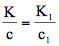(27)

and the quantity of the reaction products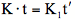does not change. By this means the slow down of the rate of time is compensated by acceleration of all processes with increasing the light velocity. As a result, all processes, including vital activity, occur in the same manner, in both frames of reference, in accordance with General Principle of Relativity.

The principle of relativity, in the most general form, including acceleration and rotation of frames of reference, was taken into account by G. Shipov in his theory of physical vacuum . Shipov's vacuum equations have allowed the General Theory of Relativity to be combined with electrodynamics and with quantum field theory. It turned out that these equations do not contain any universal constants such as light velocity, Planck's constant or elementary electrical charge. Certain combinations of these constants should be introduced into the equations. Using a certain velocity of light, c, Shipov determines a density of matter as following :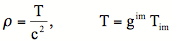(28)

where Tim is the energy-momentum tensor, gim is the metric tensor. Transformation of the coordinate frame of reference (22) conserves the value of T, so the density of matter is inversely proportional to the square of light velocity, in accordance with our assumption (23). So, an existence of material systems with a different velocity of light does not contradict the General Principle of Relativity used in the theory of physical vacuum .

## Existence of the Second Body

According to many religious doctrines, and in the views of many parapsychologists, mediums and extrasensors, we possess a second body, or soul, which leaves our physical body at the moment of death. Some people have recounted that they saw themselves from above while in the state of clinical death.

Let us assume that we really possess a second body that exists in the parallel ethereal world. Let us also assume that this second body is an exact (or almost exact) copy of our first, "physical" body: it consists of analogous atoms, molecules, cells, organs, a brain and nervous systems. What should be the mass of this body? According to eq.(10),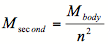(29)

There is some evidence that at the moment of death the weight of the human body decreases by several grams. If we associate this decrease with the release of the second body, it can be used to evaluate n. For example, if Mbody=80kg and ΔM=Msecond=2g,. Unfortunately, the data for weight decrease is not reliable because the weight may decrease for a number of different reasons. But it gives us a principal possibility to evaluate n and correspondingly the speed of light in the ethereal world.

The statement that the second body is tightly bound to the "physical" body and leaves it during death leads to the following conclusions:

 1) Our two worlds may interact: a) change in weight manifests gravitational interaction of an ethereal body with the Earth; b) attractive interaction between our ethereal and "physical" bodies, Fet-ph, exists; 2) The force of interaction Fet-ph depends on vital functions of our body: when they cease or fall off substantially, this force vanishes (or weakens, as in the case of clinical death); 3) This is a long-range interaction: the second body may return to the "physical" one if the latter resumes its vital functions.

The existence of this force of interaction can be connected with energy flows both in our physical body and in the second body while we are alive. However, this will be the subject of future work.

## Conclusions

The invisible part of the universe has a physical structure similar to the world we sense: the matter is built from atoms, which, in turn, are constructed from nucleons and electrons, with the same elementary electrical charge. The size, structure and energy spectrum of ethereal atoms and presently detected atoms are the same.

The ethereal world is invisible to us because of different "universal" constants such as the speed of light, c, and Planck's constant, h, while their product, c×h, which determines the elementary charge, is conserved. The ethereal speed of light is much higher than the one in our world, and Planck's constant is much smaller. This leads to higher frequencies in the ethereal elementary particles, and in particular, photons, which realize the electromagnetic interaction between atoms. Our atoms cannot absorb ethereal photons, and the ethereal world remains invisible to us.

There apparently exists gravitational interaction between the ethereal world and our world, but this interaction is very small because of the very small masses of ethereal bodies.

## References

 1 Sir William Crookes FRS, Researches into the Phenomena of Spiritualism, Two Worlds Publishing Company Ltd, 1904. 2 Sir Oliver Lodge FRS, The Mode of Future Existence, 1933 Lecture. 3 Michael Roll, The Scientific Proof of Survival after Death - www.cfpf.org.uk 4 Ronald Pearson, Consciousness as a Sub-Quantum Phenomena, Frontier Perspectives, the magazine of The Center for Frontier Perspectives at Temple University. Volume 6, Number 2. Spring/Summer 1997. 5 Shipov G. I., Theory of physical vacuum, "Nauka", Moscow, 1997 6 Madelung B. Zetshr.Phys.,1926, Bd.40, 332 7 Alekseev B.V., Abakumov A.M., DAN USSR, 1982, v.262, No.5, p.1100.
 Copyright © 1980-2005 The Campaign for Philosophical Freedom Unless otherwise indicated, all content is free to reproduce and rebroadcast for non-commercial use. Please include the credit: <> September 2005

 You are visiting our website:  W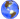rld ITC.org       To reach our homepage click here please.